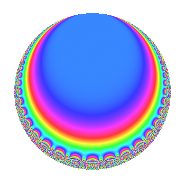Properties

 Label 128.4.b.bLevel 128 Weight 4 Character orbit 128.b Analytic conductor 7.552 Analytic rank 0 Dimension 2 CM disc. -8 Inner twists 4

Related objects

Newspace parameters

 Level: $$N$$ = $$128 = 2^{7}$$ Weight: $$k$$ = $$4$$ Character orbit: $$[\chi]$$ = 128.b (of order $$2$$ and degree $$1$$)

Newform invariants

 Self dual: No Analytic conductor: $$7.55224448073$$ Analytic rank: $$0$$ Dimension: $$2$$ Coefficient field: $$\Q(\sqrt{-2})$$ Coefficient ring: $$\Z[a_1, a_2, a_3]$$ Coefficient ring index: $$2$$ Sato-Tate group: $\mathrm{U}(1)[D_{2}]$

$q$-expansion

Coefficients of the $$q$$-expansion are expressed in terms of $$\beta = 2\sqrt{-2}$$. We also show the integral $$q$$-expansion of the trace form.

 $$f(q)$$ $$=$$ $$q$$ $$+ \beta q^{3}$$ $$+ 19 q^{9}$$ $$+O(q^{10})$$ $$q$$ $$+ \beta q^{3}$$ $$+ 19 q^{9}$$ $$+ 25 \beta q^{11}$$ $$-90 q^{17}$$ $$+ 45 \beta q^{19}$$ $$+ 125 q^{25}$$ $$+ 46 \beta q^{27}$$ $$-200 q^{33}$$ $$+ 522 q^{41}$$ $$-171 \beta q^{43}$$ $$-343 q^{49}$$ $$-90 \beta q^{51}$$ $$-360 q^{57}$$ $$-115 \beta q^{59}$$ $$-387 \beta q^{67}$$ $$+ 430 q^{73}$$ $$+ 125 \beta q^{75}$$ $$+ 145 q^{81}$$ $$+ 241 \beta q^{83}$$ $$-1026 q^{89}$$ $$+ 1910 q^{97}$$ $$+ 475 \beta q^{99}$$ $$+O(q^{100})$$ $$\operatorname{Tr}(f)(q)$$ $$=$$ $$2q$$ $$\mathstrut +\mathstrut 38q^{9}$$ $$\mathstrut +\mathstrut O(q^{10})$$ $$2q$$ $$\mathstrut +\mathstrut 38q^{9}$$ $$\mathstrut -\mathstrut 180q^{17}$$ $$\mathstrut +\mathstrut 250q^{25}$$ $$\mathstrut -\mathstrut 400q^{33}$$ $$\mathstrut +\mathstrut 1044q^{41}$$ $$\mathstrut -\mathstrut 686q^{49}$$ $$\mathstrut -\mathstrut 720q^{57}$$ $$\mathstrut +\mathstrut 860q^{73}$$ $$\mathstrut +\mathstrut 290q^{81}$$ $$\mathstrut -\mathstrut 2052q^{89}$$ $$\mathstrut +\mathstrut 3820q^{97}$$ $$\mathstrut +\mathstrut O(q^{100})$$

Character Values

We give the values of $$\chi$$ on generators for $$\left(\mathbb{Z}/128\mathbb{Z}\right)^\times$$.

 $$n$$ $$5$$ $$127$$ $$\chi(n)$$ $$-1$$ $$1$$

Embeddings

For each embedding $$\iota_m$$ of the coefficient field, the values $$\iota_m(a_n)$$ are shown below.

For more information on an embedded modular form you can click on its label.

Label $$\iota_m(\nu)$$ $$a_{2}$$ $$a_{3}$$ $$a_{4}$$ $$a_{5}$$ $$a_{6}$$ $$a_{7}$$ $$a_{8}$$ $$a_{9}$$ $$a_{10}$$
65.1
 − 1.41421i 1.41421i
0 2.82843i 0 0 0 0 0 19.0000 0
65.2 0 2.82843i 0 0 0 0 0 19.0000 0
 $$n$$: e.g. 2-40 or 990-1000 Significant digits: Format: Complex embeddings Normalized embeddings Satake parameters Satake angles

Inner twists

Char. orbit Parity Mult. Self Twist Proved
1.a Even 1 trivial yes
8.d Odd 1 CM by $$\Q(\sqrt{-2})$$ yes
4.b Odd 1 yes
8.b Even 1 yes

Hecke kernels

This newform can be constructed as the intersection of the kernels of the following linear operators acting on $$S_{4}^{\mathrm{new}}(128, [\chi])$$:

 $$T_{3}^{2}$$ $$\mathstrut +\mathstrut 8$$ $$T_{7}$$